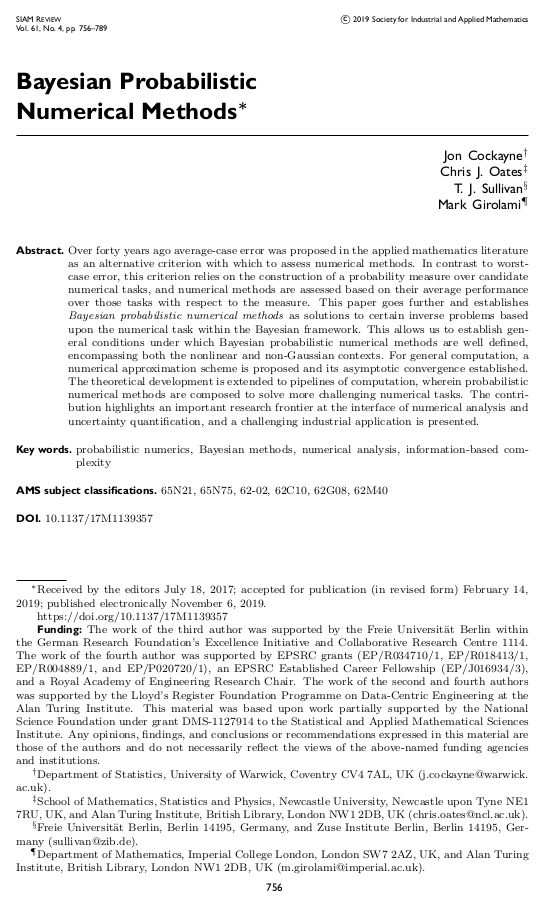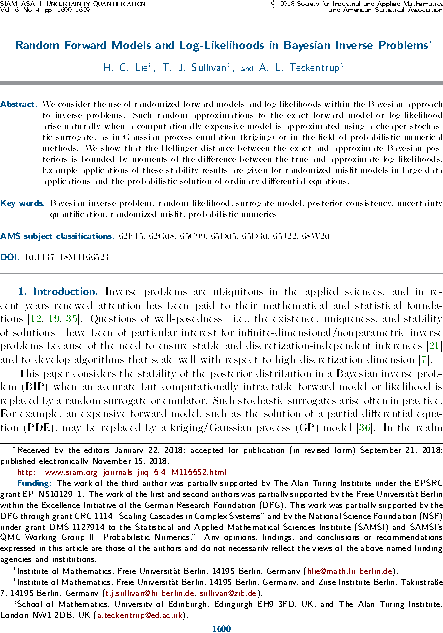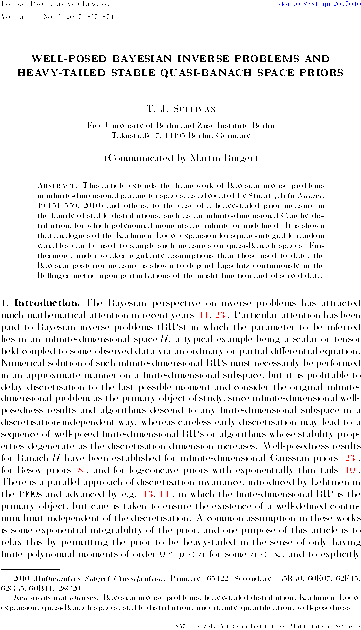# Tim Sullivan

### #bayesian

Clear Search### Linear conditional expectation in Hilbert space

Ilja Klebanov, Björn Sprungk, and I have just uploaded a preprint of our recent work “The linear conditional expectation in Hilbert space” to the arXiv. In this paper, we study the best approximation $$\mathbb{E}^{\mathrm{A}}[U|V]$$ of the conditional expectation $$\mathbb{E}[U|V]$$ of an $$\mathcal{G}$$-valued random variable $$U$$ conditional upon a $$\mathcal{H}$$-valued random variable $$V$$, where “best” means $$L^{2}$$-optimality within the class $$\mathrm{A}(\mathcal{H}; \mathcal{G})$$ of affine functions of the conditioning variable $$V$$. This approximation is a powerful one and lies at the heart of the Bayes linear approach to statistical inference, but its analytical properties, especially for $$U$$ and $$V$$ taking values in infinite-dimensional spaces $$\mathcal{G}$$ and $$\mathcal{H}$$, are only partially understood — which this article aims to rectify.

Abstract. The linear conditional expectation (LCE) provides a best linear (or rather, affine) estimate of the conditional expectation and hence plays an important rôle in approximate Bayesian inference, especially the Bayes linear approach. This article establishes the analytical properties of the LCE in an infinite-dimensional Hilbert space context. In addition, working in the space of affine Hilbert–Schmidt operators, we establish a regularisation procedure for this LCE. As an important application, we obtain a simple alternative derivation and intuitive justification of the conditional mean embedding formula, a concept widely used in machine learning to perform the conditioning of random variables by embedding them into reproducing kernel Hilbert spaces.

Published on Friday 28 August 2020 at 09:00 UTC #preprint #tru2 #bayesian #rkhs #mean-embedding #klebanov #sprungk### Bayesian probabilistic numerical methods in SIAM Review

The 2019 Q4 issue of SIAM Review will carry an article by Jon Cockayne, Chris Oates, Mark Girolami, and myself on the Bayesian formulation of probabilistic numerical methods, i.e. the interpretation of deterministic numerical tasks such as quadrature and the solution of ordinary and partial differential equations as (Bayesian) statistical inference tasks.

J. Cockayne, C. J. Oates, T. J. Sullivan, and M. Girolami. “Bayesian probabilistic numerical methods.” SIAM Review 61(4):756–789, 2019. doi:10.1137/17M1139357

Abstract. Over forty years ago average-case error was proposed in the applied mathematics literature as an alternative criterion with which to assess numerical methods. In contrast to worst-case error, this criterion relies on the construction of a probability measure over candidate numerical tasks, and numerical methods are assessed based on their average performance over those tasks with respect to the measure. This paper goes further and establishes Bayesian probabilistic numerical methods as solutions to certain inverse problems based upon the numerical task within the Bayesian framework. This allows us to establish general conditions under which Bayesian probabilistic numerical methods are well defined, encompassing both the nonlinear and non-Gaussian contexts. For general computation, a numerical approximation scheme is proposed and its asymptotic convergence established. The theoretical development is extended to pipelines of computation, wherein probabilistic numerical methods are composed to solve more challenging numerical tasks. The contribution highlights an important research frontier at the interface of numerical analysis and uncertainty quantification, and a challenging industrial application is presented.

Published on Thursday 7 November 2019 at 07:00 UTC #publication #bayesian #siam-review #prob-num #cockayne #girolami #oates### Random Bayesian inverse problems in JUQ

The article “Random forward models and log-likelihoods in Bayesian inverse problems” by Han Cheng Lie, Aretha Teckentrup, and myself has now appeared in its final form in the SIAM/ASA Journal on Uncertainty Quantification, volume 6, issue 4. This paper considers the effect of approximating the likelihood in a Bayesian inverse problem by a random surrogate, as frequently happens in applications, with the aim of showing that the perturbed posterior distribution is close to the exact one in a suitable sense. This article considers general randomisation models, and thereby expands upon the previous investigations of Stuart and Teckentrup (2018) in the Gaussian setting.

H. C. Lie, T. J. Sullivan, and A. L. Teckentrup. “Random forward models and log-likelihoods in Bayesian inverse problems.” SIAM/ASA Journal on Uncertainty Quantification 6(4):1600–1629, 2018. doi:10.1137/18M1166523

Abstract. We consider the use of randomised forward models and log-likelihoods within the Bayesian approach to inverse problems. Such random approximations to the exact forward model or log-likelihood arise naturally when a computationally expensive model is approximated using a cheaper stochastic surrogate, as in Gaussian process emulation (kriging), or in the field of probabilistic numerical methods. We show that the Hellinger distance between the exact and approximate Bayesian posteriors is bounded by moments of the difference between the true and approximate log-likelihoods. Example applications of these stability results are given for randomised misfit models in large data applications and the probabilistic solution of ordinary differential equations.

Published on Monday 10 December 2018 at 12:00 UTC #publication #bayesian #inverse-problems #juq #prob-num #lie #teckentrup### Random Bayesian inverse problems

Han Cheng Lie, Aretha Teckentrup, and I have just uploaded a preprint of our latest article, “Random forward models and log-likelihoods in Bayesian inverse problems”, to the arXiv. This paper considers the effect of approximating the likelihood in a Bayesian inverse problem by a random surrogate, as frequently happens in applications, with the aim of showing that the perturbed posterior distribution is close to the exact one in a suitable sense. This article considers general randomisation models, and thereby expands upon the previous investigations of Stuart and Teckentrup (2018) in the Gaussian setting.

Abstract. We consider the use of randomised forward models and log-likelihoods within the Bayesian approach to inverse problems. Such random approximations to the exact forward model or log-likelihood arise naturally when a computationally expensive model is approximated using a cheaper stochastic surrogate, as in Gaussian process emulation (kriging), or in the field of probabilistic numerical methods. We show that the Hellinger distance between the exact and approximate Bayesian posteriors is bounded by moments of the difference between the true and approximate log-likelihoods. Example applications of these stability results are given for randomised misfit models in large data applications and the probabilistic solution of ordinary differential equations.

Published on Tuesday 19 December 2017 at 08:30 UTC #preprint #bayesian #inverse-problems #lie #teckentrup### Heavy-tailed stable priors in Inverse Problems and Imaging

The final version of “Well-posed Bayesian inverse problems and heavy-tailed stable quasi-Banach space priors” has now been published online in Inverse Problems and Imaging; the print version will be available in October.

T. J. Sullivan. “Well-posed Bayesian inverse problems and heavy-tailed stable quasi-Banach space priors.” Inverse Problems and Imaging 11(5):857–874, 2017. doi:10.3934/ipi.2017040

Published on Wednesday 19 July 2017 at 15:30 UTC #publication #bayesian #inverse-problems #cauchy-distribution #inverse-problems-and-imaging